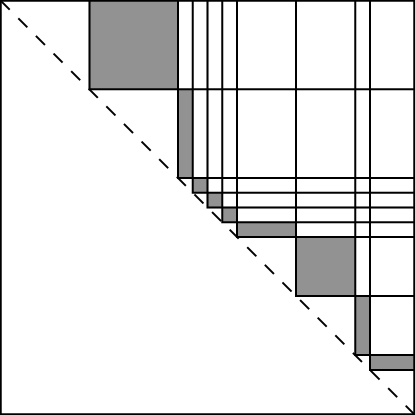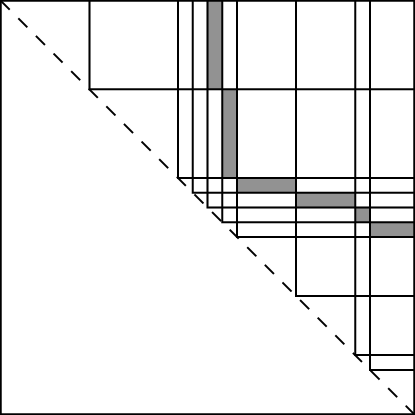Graded algebras are the path way between general algebraic structures and tensors. Suppose we have an algebriac structure $A$ and an index set $M$ which identifies a direct product decomposition

$A=\bigoplus_{s:M}A_s.$

Often graded algebras arise from filters. You can see more about that in the filters package.

So the graded algebra assumption states that each $A_s$ is an abelian group and the following.

$A_s*A_t \leq A_{s+t}.$

This sets up a number tensors, for example bilinear maps (3-tensors)

$A_s\times A_t\rightarrowtail A_{s+t}$

but also tensors of general valence such as

$A_{s_{\ell}}\times \cdots \times A_{s_1}\rightarrowtail A_{\sum_i s_i}.$

In the graded algebra project we focus on isomorphism by searching out sections of $A$ that minimize the search. If these cannot be found we start a process of breaking up homogeneous components $A_s$ into smaller parts by refining the grading. Refinement is carried out by our filters package.

Here is an illustration of a bad layer, because its total surface area is larger.By refining and scanning the other layers we can find a potentially small surface area layer.The ability to testing isomorphism from the middle is described in [BOW]. Further strategies for refinement were dealt with in [BGLQW].

• [BOW] Isomorphism testing of Graded algebras, P.A. Brooksbank, E.A. O’Brien, J.B. Wilson, Trans. Amer. Math. Soc. 372 (11) 2019, 8067–8090. arXiv:1708.08873

• [BGLQW] Incorporating Weisfeiler-Leman into algorithms for group isomorphism, Peter A. Brooksbank, Joshua A. Grochow, Yinan Li, Youming Qiao, James B. Wilson arXiv:1905.02518P.A. Brooksbank, J. Maglione, J. B. Wilson